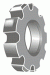I have forgotten

•http://facebook.com/
•https://www.google.com/accounts/o8/id
•https://me.yahoo.com27 May 11, 7:56AM
$\frac{1-\sin(x^2)}{x}$
<img src="http://latex.codecogs.com/gif.latex?\frac{1-\sin(x^2)}{x}" alt="\frac{1-\sin(x^2)}{x}" />